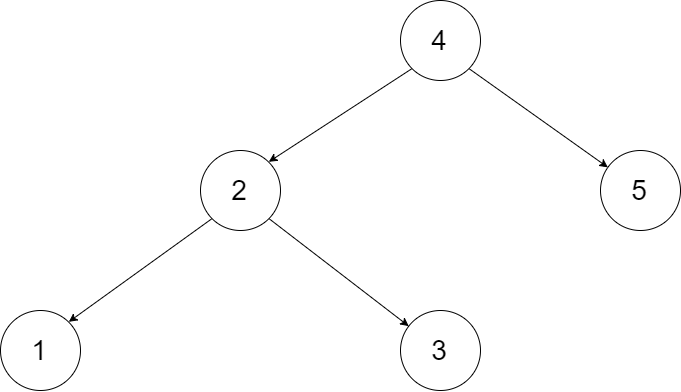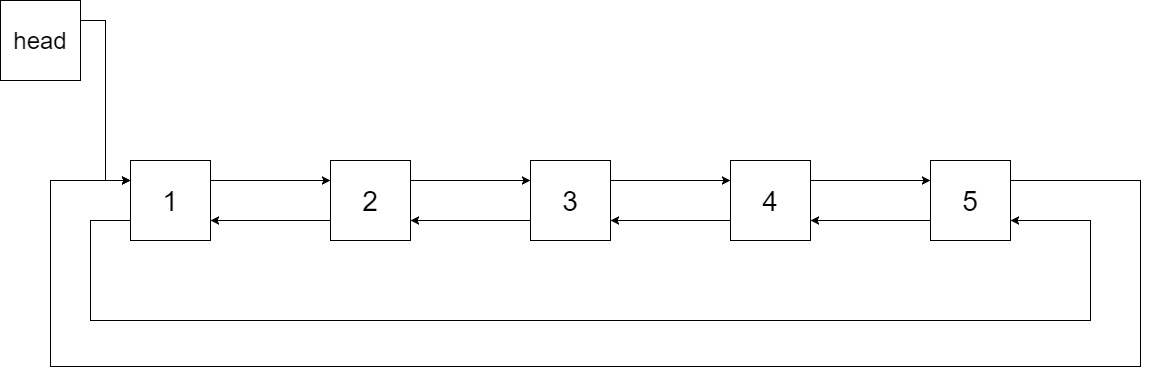##### Welcome to Subscribe On Youtube

Formatted question description: https://leetcode.ca/all/426.html

# 426. Convert Binary Search Tree to Sorted Doubly Linked List

Medium

## Description

Convert a Binary Search Tree to a sorted Circular Doubly-Linked List in place.

You can think of the left and right pointers as synonymous to the predecessor and successor pointers in a doubly-linked list. For a circular doubly linked list, the predecessor of the first element is the last element, and the successor of the last element is the first element.

We want to do the transformation in place. After the transformation, the left pointer of the tree node should point to its predecessor, and the right pointer should point to its successor. You should return the pointer to the smallest element of the linked list.

Example 1:Input: root = [4,2,5,1,3]Output: [1,2,3,4,5]

Explanation: The figure below shows the transformed BST. The solid line indicates the successor relationship, while the dashed line means the predecessor relationship.Example 2:

Input: root = [2,1,3]

Output: [1,2,3]

Example 3:

Input: root = []

Output: []

Explanation: Input is an empty tree. Output is also an empty Linked List.

Example 4:

Input: root = 

Output: 

Constraints:

• -1000 <= Node.val <= 1000
• Node.left.val < Node.val < Node.right.val
• All values of Node.val are unique.
• 0 <= Number of Nodes <= 2000

## Solution

If the root is null, simply return the root. If there is only one node in the binary search tree, then let the root’s predecessor and successor both point to the root itself, and return the root.

Use the idea of the solution to problem 94. Consider inorder traversal of the binary search tree, which contains all the elements sorted in ascending order.

However, this problem does not require returning a list of inorder traversal result. Therefore, when doing inorder traversal, maintain a previous node, which is initially null. Each time a node is met, make the previous node’s successor be the current node, and make the current node’s predecessor be the previous node.

After all the nodes are visited, all the relations between each pair of adjacent nodes are maintained. Since the list is required to be circular, make the head node’s predecessor be the tail node, and make the tail node’s successor be the head node. Finally, return the head node.

• /*
// Definition for a Node.
class Node {
public int val;
public Node left;
public Node right;

public Node() {}

public Node(int _val) {
val = _val;
}

public Node(int _val,Node _left,Node _right) {
val = _val;
left = _left;
right = _right;
}
};
*/
class Solution {
public Node treeToDoublyList(Node root) {
if (root == null)
return root;
if (root.left == null && root.right == null) {
root.left = root;
root.right = root;
return root;
}
Node head = root, tail = root;
while (tail.right != null)
tail = tail.right;
inorderTraversal(root);
}

public void inorderTraversal(Node root) {
Stack<Node> stack = new Stack<Node>();
Node node = root;
Node prevNode = null;
while (!stack.isEmpty() || node != null) {
while (node != null) {
stack.push(node);
node = node.left;
}
Node visitNode = stack.pop();
visitNode.left = prevNode;
if (prevNode != null)
prevNode.right = visitNode;
node = visitNode.right;
prevNode = visitNode;
}
}
}

• // OJ: https://leetcode.com/problems/convert-binary-search-tree-to-sorted-doubly-linked-list/
// Time: O(N)
// Space: O(H)
class Solution {
public:
Node* treeToDoublyList(Node* root) {
if (!root) return NULL;
auto left = treeToDoublyList(root->left), right = treeToDoublyList(root->right);
root->left = left ? left->left : (right ? right->left : root);
root->right = right ? right : (left ? left : root);
if (left) {
left->left->right = root;
left->left = right ? right->left : root;
}
if (right) {
right->left->right = left ? left : root;
right->left = root;
}
return left ? left : root;
}
};

• """
# Definition for a Node.
class Node:
def __init__(self, val, left=None, right=None):
self.val = val
self.left = left
self.right = right
"""

class Solution:
def treeToDoublyList(self, root: 'Optional[Node]') -> 'Optional[Node]':
def dfs(root):
if root is None:
return
dfs(root.left)
if prev:
prev.right = root
root.left = prev
else:
prev = root
dfs(root.right)

if root is None:
return None
dfs(root)


• /**
* Definition for a Node.
* type Node struct {
*     Val int
*     Left *Node
*     Right *Node
* }
*/

func treeToDoublyList(root *Node) *Node {
if root == nil {
return root
}

var dfs func(root *Node)
dfs = func(root *Node) {
if root == nil {
return
}
dfs(root.Left)
if prev != nil {
prev.Right = root
root.Left = prev
} else {
}
prev = root
dfs(root.Right)
}
dfs(root)
}

• /**
* // Definition for a Node.
* function Node(val, left, right) {
*      this.val = val;
*      this.left = left;
*      this.right = right;
*  };
*/

/**
* @param {Node} root
* @return {Node}
*/
var treeToDoublyList = function (root) {
if (!root) return root;
let prev = null;

function dfs(root) {
if (!root) return;
dfs(root.left);
if (prev) {
prev.right = root;
root.left = prev;
} else {
}
prev = root;
dfs(root.right);
}
dfs(root);